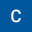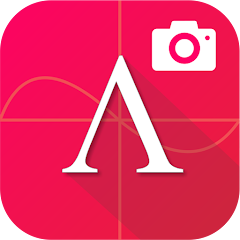# ALGEBRATOR-step-by-step solver

In-app purchases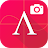3.2
3.69K reviews
1M+Everyone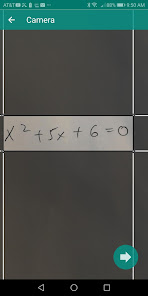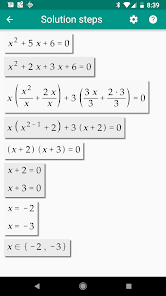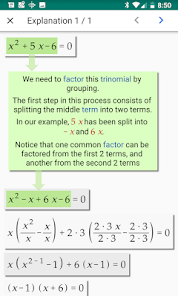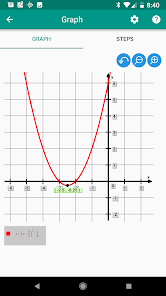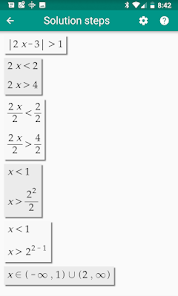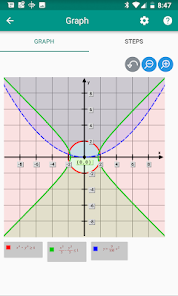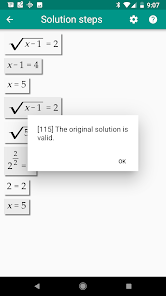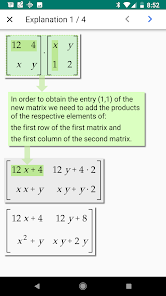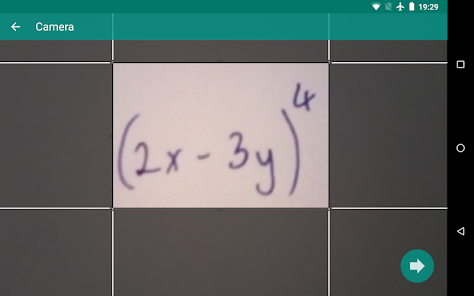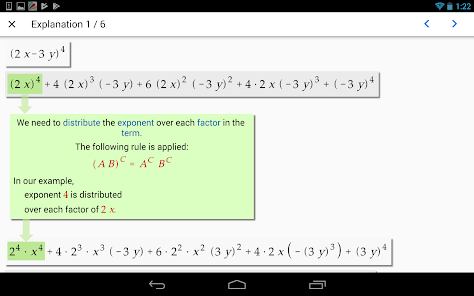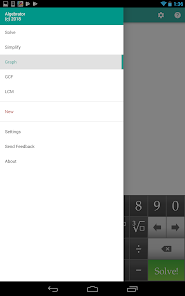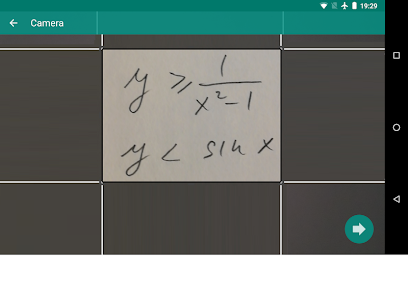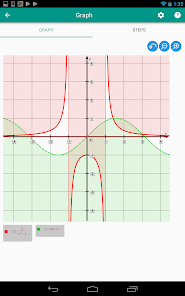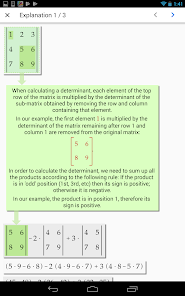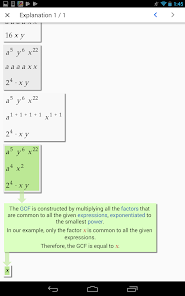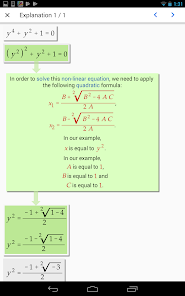Take a photo of your math problem and Algebrator will solve and explain it!
We have been helping high-school and college students conquer math for more than a decade. Our camera math solver shows all steps of the solution as well as explanations to any math textbook problem you enter. This camera calculator app solves math homework problems just like your teacher! Explanations will tell you not only which algebra rule is being used, but also how it is applied in your particular math problem. Snap the problem picture or enter it via user friendly math keyboard to get instant steps and solution!

Fraction calculator will show steps to add, subtract and multiply fractions, as well as complex fraction division. After reducing fractions or rational numbers to simplest terms, fraction to decimal calculator will assist you with converting fractions to percentages. Lowest common denominator (lcm, lcd) calculator will help make equivalent fractions. Simplifying fractions also includes rationalizing denominators and numerators.
Graphing calculator will graph lines, horizontal and vertical asymptotes, x and y intercepts. It will produce graphs of quadratic equations, functions and parabola equations, linear equations (lines) and inequalities, trig. functions (e.g. cos graph) and curves such as hyperbola and circle equations. The graphing calculator math app will also show fractions on the number line, find the slope of a line as well as a parabola vertex. After placing polynomials in standard form, it will graph any polynomial function. Many other functions such as logarithmic, exponential and absolute value can also be graphed.
Equations and inequalities can be solved by several math equation solvers (e.g. quadratic equation calculator, linear equations calculator and system of equations solver (solving by substitution and addition)). Quadratic calculator will put a quadratic equation in standard form and then either use quadratic formula or solve by factoring. Logarithmic, rational, exponential, linear and quadratic equation solver will solve for x or any other math variable. Systems of linear equations and inequalities will be graphed to produce visually appealing math solution. Linear inequalities, polynomial equations, absolute value equations and inequalities are all solved by our algebra solver that shows work just like your teacher.
Factoring solver can factor polynomials, trinomials and binomials. It will factor quadratic equation so that it can be solved by the equation solver. Many different factoring methods such as factoring by grouping, difference of two squares, difference of two cubes, sum of two cubes are used. Greatest Common Factor calculator and perfect square trinomials are also used in the polynomial factoring calculator.
Trigonometry solver simplifies algebraic expressions with trig functions (such as sin or cos). It knows special values on trig unit circle (e.g. cos 0, sin 0, cos 1, sin 1, cos 30, sin 30, cos 45, sin 45, cos 90, sin 90). Sin cos tan and other trig functions can be simplified and graphed.
Exponents calculator applies exponent power rules and laws of exponents. It multiplies and divides fractional and negative exponents, using the properties of exponents. It also knows logarithm rules to apply to logarithmic functions.
Simplify expression calculator can simplify any algebraic or math expression, showing you all the step in the process. This includes complex numbers and imaginary numbers that are handled by calculator for complex numbers.
Radical simplifier includes cube and square root solver.
Square root properties are used while solving radical equations and simplifying radical expressions.
Matrix solver can multiply matrices, find inverse matrix and perform other matrix operations. Matrix determinant calculator can find any determinant value.
If you need help with math then our free math app is the here for you. Softmath has provided help with Algebra 1, Algebra 2 and College Algebra since 1990.
Updated on
Aug 28, 2023

## Data safety

Safety starts with understanding how developers collect and share your data. Data privacy and security practices may vary based on your use, region, and age. The developer provided this information and may update it over time.No data shared with third partiesThis app may collect these data types
Device or other IDs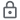Data is encrypted in transit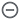Data can’t be deleted

## Ratings and reviews

3.3
3.56K reviews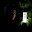Ahanaf Alam
July 16, 2023
My experience for this app is very good. It is a very helpful app for high schoolers. This is the best algebra calculator ever. This calculator even has a free version for those who can't pay the subscription. You just have to reach out to them. So I think this app deserves a 5 star rating.😇😀
15 people found this review helpful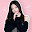Ice girl
June 18, 2023
Although this is a great app for secondary math students like me to solve questions involving surds but after searching for one question, then the rest of the questions the app only provides answers and only have part of the solutions, then you will have to pay for it before having full worked solutions. Overall, this app not only have answers but also have solutions so it is a wonderful app for math surds.
19 people found this review helpful
Softmath
June 30, 2023